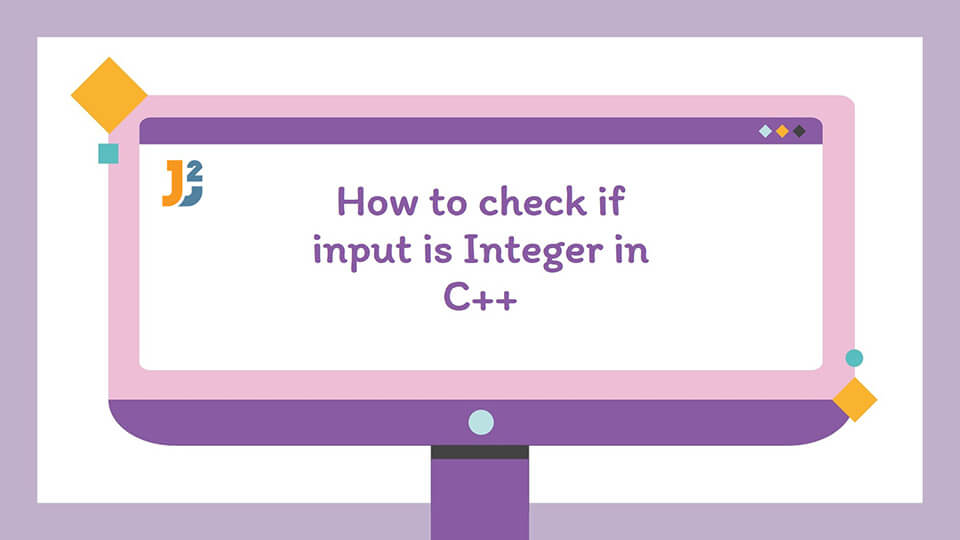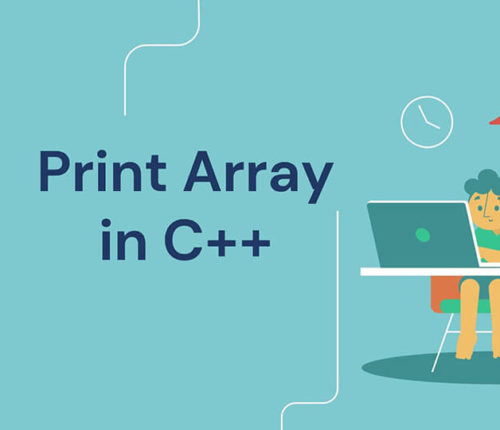# Check If Input Is Integer In C++C++ is a powerful and popular programming language and we use it widely in coding applications. It provides us with a number of powerful means to check the input given by users. In this article, we will discuss how we can check whether the input given by users is an integer or not.

## How to Check Whether the Input Is an Integer in C++?

When we need to perform operations on integers, it is important to check the input so that the operation is performed smoothly and does not produce undesired results. We can have a number of such applications especially in applications like calculators.

Once the input is taken into a string or an array of characters we can check it for its type. For this, we can traverse the input from left to right and check whether each character is a digit or not. We can use two different methods for this.

In the first method, we can use the `isdigit()` function which is implemented in the C++ library to check whether the character is a digit or not.

In the second method, we can manually check each character by comparing the ASCII value of the character with the ASCII value of the digits. In this way, we will be able to check our input. I have given the code of both of the methods separately but both the approaches are similar so I have written the approach only once. Interestingly, the code works for both positive as well as negative integer inputs.

## Check Whether the Input Is an Integer Using the isdigit() Function in C++

The isdigit() function is an inbuilt library function that takes a character as the input argument and returns a boolean value based on the character. If the character is found to be a digit it returns true otherwise it returns false. To check for integer input using the `isdigit()` function, we will follow the following steps.

• First, we will define a string variable to store the user input.
• Next, we will ask the user for the input through standard input.
• To check if the input is integer or not, we will define a function named `checkInteger()`. It will take the string as an input argument, and check whether the input string is an integer or not. It returns true if the input is an integer otherwise returns false.
• Inside the `checkInteger()` function:
•  First, we will check if the input string is empty. If yes, It means that the input string is not an integer. Hence, we will return False.
• Otherwise, we will check if the first character is a minus sign (-) so that we can check if the input is a negative integer. If we find a negative sign we have to skip it.
• After checking for the negative sign,  we will use a for loop and the `isdigit()` function. For each character in the input string, we will check if it is a decimal digit or not using the` isdigit()` function. If there exists any character that isn’t an integer, the `isdigit()` function will return False. Hence, the `checkInteger()` function will return False denoting that the input string is not an integer.
• If there are only decimal digits in the string, the control reaches to the end of the `checkInteger()` function out of the loop. In such a case, we return true denoting that the input string is an integer.
• After the function `checkInteger()` finishes its execution, we return to the `main()` function.
• In the `main()` function, we check if the function `checkInteger() `returns True. If yes, we print output “Yes” denoting that the input string is an integer. Otherwise we print “No”.

Following is the implementation of the above approach to check if an input is an integer in C++.

Output:

## Check Whether the Input Is an Integer Using the Ascii Values in C++

In this approach, instead of the `isdigit()` function, we can check whether a character is a digit or not by comparing the ASCII value of the character with the ASCII value of known digits.  Here, we will use the ASCII value of the character and compare it with the known ASCII values of the integer characters (from ‘0’ till ‘9’). If the character is found to be outside the range of ASCII values from ‘0’ till ‘9’, the character is not an integer.

For implementing this approach, we can modify the` checkInteger()` function and insert the code for the above logic instead of the `isdigit()` function as follows.

Output:

## Conclusion

We come across multiple instances where we need to check whether the input is an integer or not while programming in C++. Before performing operations, we need to check the type of input so that the operations can be performed efficiently and the code does not produce undesired results. In this article, we have seen two different approaches to check if an input is an integer or not in C++. Both methods have approximately the same performance. You can use any of them to check if the user input is an integer or not in C++.

Happy Learning!

## Related Posts

•23 April

### How to initialize an Array in Constructor in C++

Table of ContentsArraysConstructors of a ClassWays to initialize an array in Constructor in C++Initialize an array in Constructor With std::fill()Initialize an array in Constructor Using for LoopInitialize an array in Constructor Using Member Initializer ListConclusion This article discusses methods of initializing an array in constructor in C++. Let us first discuss arrays and constructors in […]

•31 March

### Write String to File in C++

Table of ContentsUsing the fstream Library to write String to File in C++Using the Insertion Operator (<<) With the fstream ObjectUsing the write() Function of the fstream LibraryUsing the fwrite() Function to write String to File in C++Conclusion The files are used to store the data permanently on a disk. This mitigates the problem of […]

•23 March

### Print Array in C++

Table of ContentsIntroduction of ArraysDifferent Methods to Print an Array in C++print array in C++ by Traversing Indicesprint array in C++ Using for_each() Functionprint array in C++ Using Range-Based for Loopprint array in C++ Using Iteratorsprint array Using ostream_iterator() Function and copy() Functionprint array Using C++17 copy() Function and make_ostream_joiner() FunctionConclusion An array is a […]

•23 March

### Print Vector in C++

Table of ContentsPrint Vector in C++ Using IndicesPrint Vector in C++ Using Range-Based for LoopPrint Vector in C++ Using for_each() FunctionPrint Vector in C++ Using IteratorPrint Vector in C++ by Overloading the Insertion Operator (<<)Print Vector in C++ Using copy() FunctionConclusion This article discusses the vector and how to print vector in C++. Vectors are […]

•15 March

### Print Map in C++

Table of ContentsMap vs Unordered MapPrint Map in C++ Using IteratorPrint Map in C++ Using Range-Based for LoopPrint Map in C++ by Overloading the ostream Insertion Operator (<<)Print Map in C++17 Using Structure Binding and Range-Based for LoopPrint Map in C++17 Using for_each() FunctionConclusionReferences Maps in C++ store the data in form of key and […]

•14 March

### Write Binary Files in C++

Table of ContentsOpening a File in Binary Mode Using fstream LibraryWriting the Binary File Using the write() Function of fstream LibraryWriting the Binary File Using the put() Function of fstream LibraryWriting the Binary File Using Insertion Operator (<<) Of fstream LibraryWriting the Structure Object to Binary File Using fstream LibraryConclusion In this post, we will […]

## Subscribe to our newletter

Get quality tutorials to your inbox. Subscribe now.